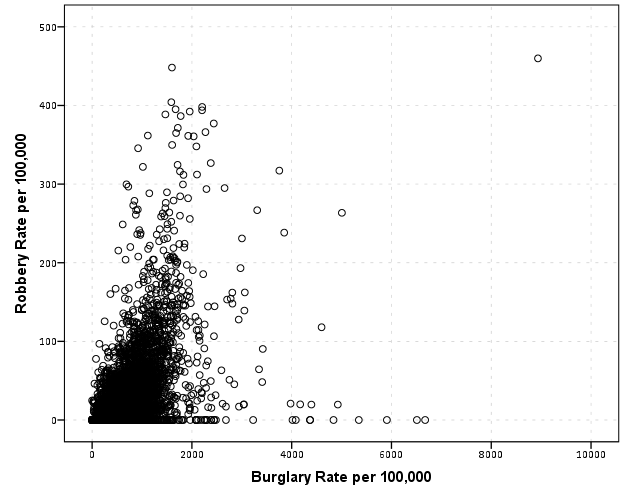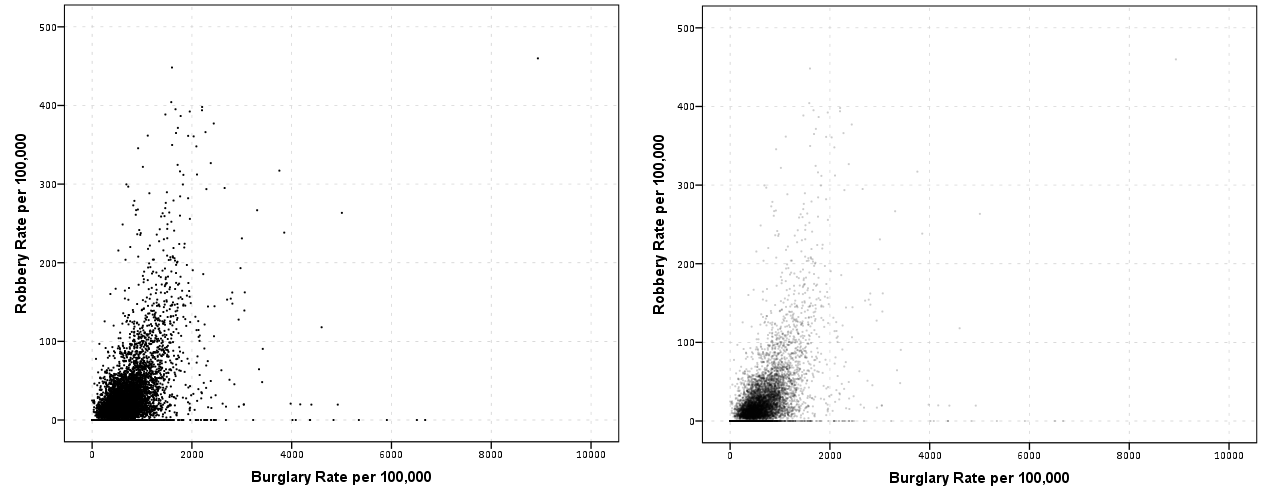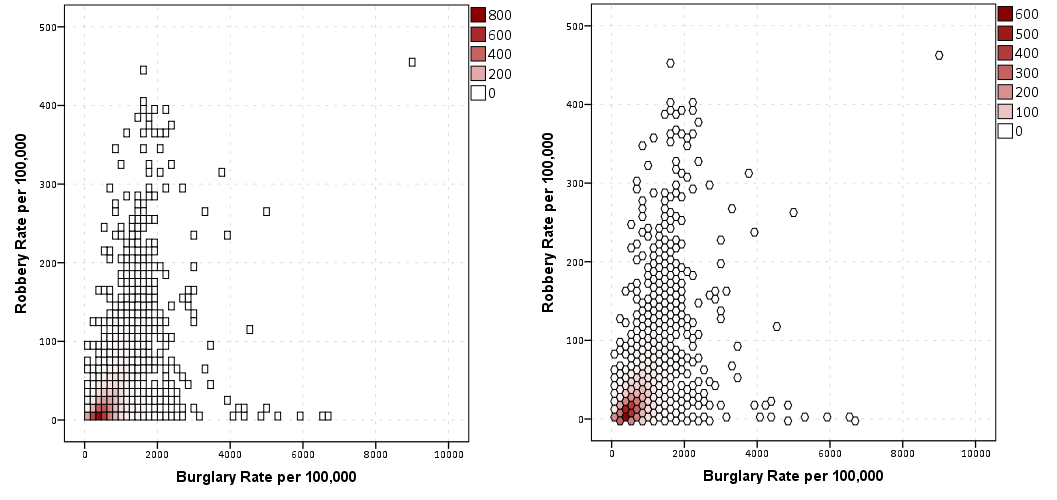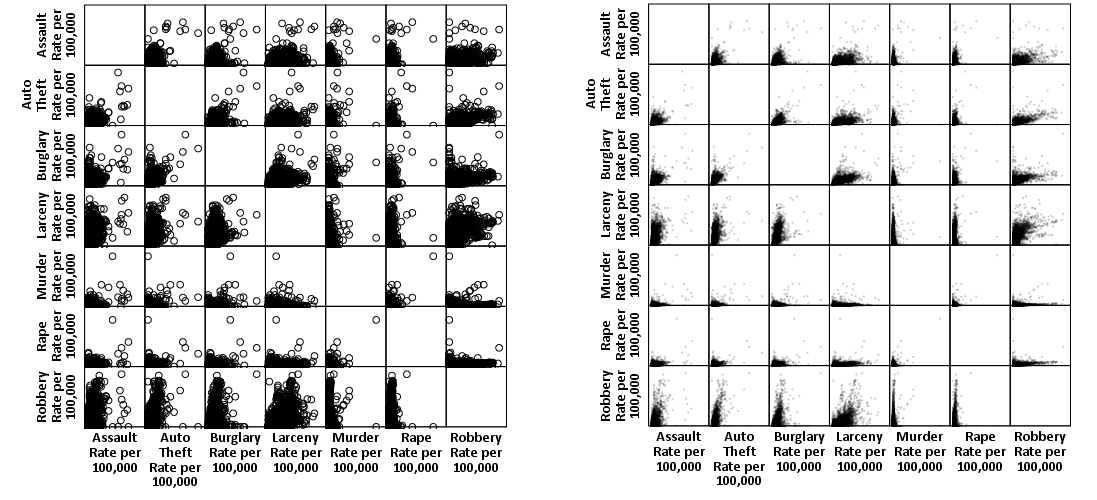# SPSS Statistics

View Only

## Visualization techniques for large N scatterplots in SPSS

####By Archive User posted Sun June 17, 2012 10:18 AM

When you have a large N scatterplot matrix, you frequently have dramatic over-plotting that prevents effectively presenting the relationship. Here I will give a few quick examples of simple ways to alter the typical default scatterplot to ease the presentation. I give examples in SPSS, although I suspect any statistical packages contains these options to alter the default scatterplot. At the end of the post I will link to SPSS code and data I used for these examples. For a brief background of the data, these are UCR index crime rates for rural counties by year in Appalachia from 1977 to 1996. This data is taken from the dataset Spatial Analysis of Crime in Appalachia, 1977-1996 posted on ICPSR (doi:10.3886/ICPSR03260.v1). While these scatterplots ignore the time dimension of the dataset, they are sufficient to demonstrate techniques to visualize big N scatterplots, as they result in over 7,000 county years to visualize.

So what is the problem with typical scatterplots for such large data? Below is an example default scatterplot in SPSS, plotting the Burglary Rate per 100,000 on the X axis versus the Robbery Rate per 100,000 on the Y axis. This uses my personal default chart template, but the problem is with the large over-plotted points in the scatter, which is the same for the default template that comes with installation.The problem with this plot is that the vast majority of the points are clustered in the lower left corner of the plot. For the most part, the graph is expanded simply due to a few outliers in both dimesions (likely due to in part hetereoskedascity that comes with rates in low population areas). While the outliers will certainly be of interest, we kind of lose the forest for the trees in this particular plot.

Two simple suggestions to the base default scatterplot are to utilize smaller points and/or makes the points semi-transparent. On the left is an example of making the points smaller, and on the right is an example utilizing semi-transparency and small points. This de-emphasizes the outlier points (which could be good or bad depending on how you look at it), but allows one to see the main point cloud and the correlation between the two rates within it. (Note: you can open up the images in a new window to see them larger)Note if you are using SPSS, to define semi-transparency you need to define it in the original GPL code (or in a chart template if you wanted), you can not do it post-hoc in the editor. You can make the points smaller in the editor, but editing charts with this many elements tends to be quite annoying, so to the extent you can specify the aesthetics in GPL I would suggest doing so. Also note making the elements smaller and semi-transparent can also be effectively utilized to visualize line plots, and I gave an example at the SPSS IBM forum recently.

Another option is to bin the elements, and SPSS has the options to either utilze rectangular bins or hexagon bins. Below is an example of each.One thing that is nice about this technique and how SPSS handles the plot, a bin is only drawn if at least one point falls within it. Thus the outliers and the one high leverage point in the plot are still readily apparent. Other ways to summarize distributions (that are currently not available in SPSS) are sunflower plots or contour plots. Sunflower plots are essentially another way to display and summarize multiple overlapping points (see Carr et al., 1987 or an example from this blog post by Analyzer Assistant). Contour plots are drawn by smoothing the distribution and then plotting lines of equal density. Here is an example of a contour plot using ggplot2 in R on the Cross Validated Q/A site).

This advice can also be extended to scatterplot matrices. In fact such advice is more important in such plots, as the relationship is shrunk in a much smaller space. I talk about this some in my post on the Cross Validated blog, AndyW says Small Multiples are the Most Underused Data Visualization when I say reducing information into key patterns can be useful.

Below on the left is an example of the default SPSS scatter plot matrix produced through the Chart Builder, and on the right after editing the GPL code to make the points smaller and semi-transparent.I very briefly experimented with adding a loess smooth line or using the binning techniques in SPSS but was not sucessful. I will have to experiment more to see if it can be effectively done in scatterplot matrices. I would like to extend some of the example corrgrams I previously made to plot the loess smoother and bivariate confidence ellipses, and you can be sure I will post the examples here on the blog if I ever get around to it.

The data and syntax used to produce the plots can be found here.

#datavisualization
#small-multiples
#SPSS
#SPSSStatistics
#Visualization
2 views

Thu July 03, 2014 02:31 PM

This message was posted by a user wishing to remain anonymous
[…] draw the points smaller and slightly transparent […]

Fri June 22, 2012 06:45 PM

Thanks for the comments Jon. Yes I agree about changing the axis to logarithms. I don't believe I put that much thought into it, but if I used log-log plots I would have to decide how to plot the cases at zero. If I were to model the data I would likely use some type of poisson regression (so I suppose representing the relationship on a log-log plot would be appropriate?) I will paste some updated examples on log-log plots when I get a chance. Maybe I will post an example also plotting prediction intervals from a poisson regression model like you see on many of the ggplot2 R package examples.

I realize that hex-bins are suggested to prevent striation in the plot, but I do not think I've seen a real problematic example. In GIS applications hex bins typically aren't available, so I am used to constructing rectangular bins/fishnet/quadrats in that domain.

I don't think I would miss sunflower plots over binning, but I would like however for contour plots to be available. I think contour plots would be useful in the scatter-matrix, and maybe easier to digest than binning.

Fri June 22, 2012 05:37 PM

Although it isn't really a large dataset issue, this particular plot would reveal more if plotted on a log-log scale.

It's worth noting that hexbinning usually works better than rectangular binning, because the later tends to overemphasize lines along the chart axes. Rectangular binning is more appropriate for histograms.

SPSS used to have sunflowers, but these were discontinued with the newer graphics engine as other techniques such as discussed above generally work better.

And don't forget, there is always sampling.